# LOG#049. Ludicrous speed.We are going to learn about the different notions of velocity that the special theory of relativity provides.

The special theory of relativity is a simple wonderful theory, but it comes with many misconceptions due to bad teaching/science divulgation. It is not easy to master the full theory of relativity without the proper mathematical background and physical insight. In the internet era where knowledge is shared, a fundamental issue is to understand things properly. There are many people who thinks they understand the theory of relativity when they don’t. Even at the academia.

Moreover, you can find many people in the blogsphere/websphere trying to sell false theories and wrong theories. It is the same like the so-called alternative medicine: they are not medicine at all. Bad science is not science, it is simply a lie and not science at all. It is religion. Science can be critized, but nobody can critize that Earth revolves around the Sun, it is common knowledge and truth. So, we can make critics to scientist, but not the scientific method and well established theories. We can try to understand better or in a novel way, but we can not deny facts and experiments. Gerard ‘t Hooft, Nobe Prize, explain it in his web page www.phys.uu.nl/~thooft/.

It is important to remark that Science revolutions come when we extend the theories we know they are correct, like special relativity and not with a full destruction of the current and well-tested theories. Newtonian relativity is a limit of General Relativity. Galilean relativity is a limit of Special Relativity. Quantum Mechanics is a limit of QFT and so on. The issue is not that. Said these words, I am quite sure that scientists and particularly physicists wish to overcome current theories with new ones. However, the process to create a new theory is not easy. Specially, if you don’t understand the traps and theories that have passed every known test till now.

What is velocity? Classically, the answer is short and very clear/neat: velocity is the rate of change of position with respect to time. It is a vector magnitude. Mathematically speaking is the quotient between the displacement vector and the time interval, or in the infinitesimal limit, the derivative of the position vector with respect to time.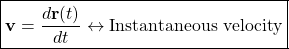In the special theory of relativity, due to the fact that time is not universal but relative we can build different notions of velocity. And it matters. There are some clear concepts from relativity you should master till now:

a) You can attach a clock to any yardstick you could physically use for measurements of space and time.

b) You must distinguish the notions of coordinate velocity (map coordinate is another commonly used notion/concept) and proper velocity. The latter is sometimes called hyperbolic (or imaginary) velocity. These two notions are caused by the presence of two “natural” elections of time: the proper time and the coordinate time.

c) Due to the previous two facts, you must also distinguish between proper acceleration and geometric acceleration. Proper-accelerations caused by the tug of external forces and geometric accelerations caused by choice of a reference frame that’s not geodesic i.e. a local reference coordinate-system that is not ”in free-fall”. Proper-accelerations are felt through their points of action e.g. through forces on the bottom of your feet. On the other hand geometric accelerations give rise to inertial forces that act on every ounce of an object’s being. They either vanish when seen from the vantage point of a local free-float frame, or give rise to non-local force effects on your mass distribution that cannot be made to disappear. Coordinate acceleration goes to zero whenever proper-acceleration is exactly canceled by that connection term, and thus when physical and inertial forces add to zero.

People who are not aware of the previous comments, don’t understand relativity and the physics behind it. They even don’t undertand what experiments and their data say.

Let me review the main magnitudes, 3-vectors and 4-vectors which the special theory of relativity studies in the next tables: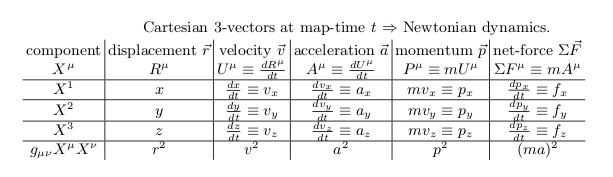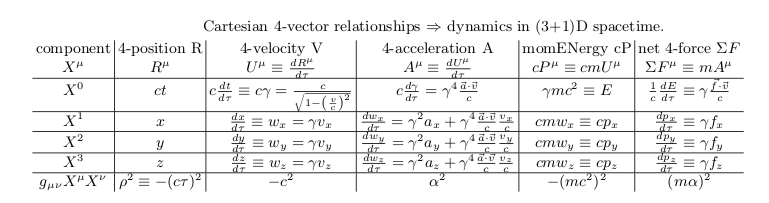The two notions of 3-velocity we do have from the special theory of relativity, i.e., from the 4-velocity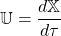,  are:

1) Coordinate velocity,: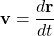It is the common notion of 3-velocity, measured from an inertial observer with respect to the coordinate time t. Note that the coordinate time is not a true invariant in SR!

2) Proper velocity (or the hyperbolic velocity/imaginary angle velocity related to it):whereis the proper time. This velocity can intuitively defined as the distance per unit traveler-time, retains many of the properties that ordinary velocity loses at high speed. In addition to these two definitions, we also have:

1)Proper-acceleration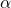, is the acceleration experienced relative to a locally co-moving free-float-frame, and it helps when we are accelerating, speeding, and in curvy space-time.

2) How some of the space-like effect of sideways ”felt” forces moves into the reference-frame’s time-domain at high speed, making the relatively unknown bound (from special relativity!)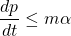With the above definitions, the relativistic momentum can be expressed in terms of coordinate velocity or proper velocity as follows: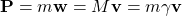where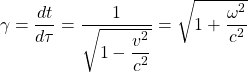is the Lorentz factor. The last equal sign in the previous equation can be easily derived from the relativistic relationship: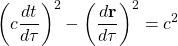and the definition of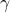above.

Thanks to the metric-equation’s assignment of a frame-invariant traveler or proper-timeto the displacement between events in context of a single map-frame of comoving yardsticks and synchronized clocks, proper velocity becomes one of three related derivatives in special relativity (coordinate velocity, proper-velocity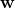, and Lorentz factor) that describe an object’s rate of travel. For unidirectional motion, in units of light speed c (i.e. c=1 if we want to) each of these is also simply related to  a traveling object’s hyperbolic velocity angle or rapidity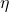by the next set of equations: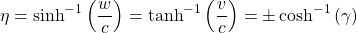The next table illustrates how the proper-velocity ofor “one map-lightyear per traveler-year” is a natural benchmark for the transition from a sub-relativistic coordinate frame to a (fake) auxiliary super-relativistic motion (in imaginary units of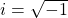). Note that the velocity angle or pseudorapidityand the proper-velocityrun from 0 to infinity and track the physical coordinate-velocity when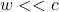. On the other hand when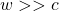, the (hyperbolic or imaginary) proper-velocity tracks Lorentz factorwhile velocity angleis logarithmic and hence increases much more slowly: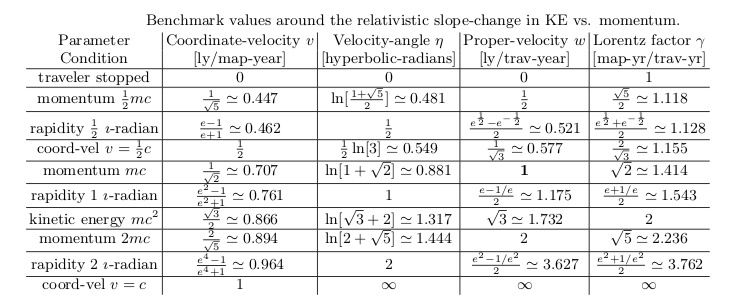LUDICROUS SPEED AND WARP SPEED

Hyperbolic velocities CAN exceed c! They can reach even the ludicrous speed of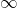when the coordinate velocity approaches c! However, you must never forget the fact that the velocity-angle/hyperbolic velocity IS imaginary in value. It is quite clear from the above table. Indeed, being somehow “trekkie” or a Sci-Fi “romantic” person, you could “define” warp-speeds as “imaginary/hyperbolic” velocities, i.e., in terms of proper velocity. In that case, you could get the correspondence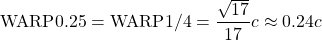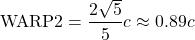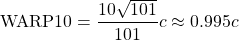In general, we can define the WARP speed asand so, the proper velocity can be expressed in terms of the warp speed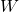in a very simple way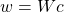. Thus, the real or coordinate velocity would be connected with warp-speed through the relativistic equation: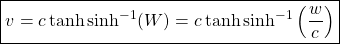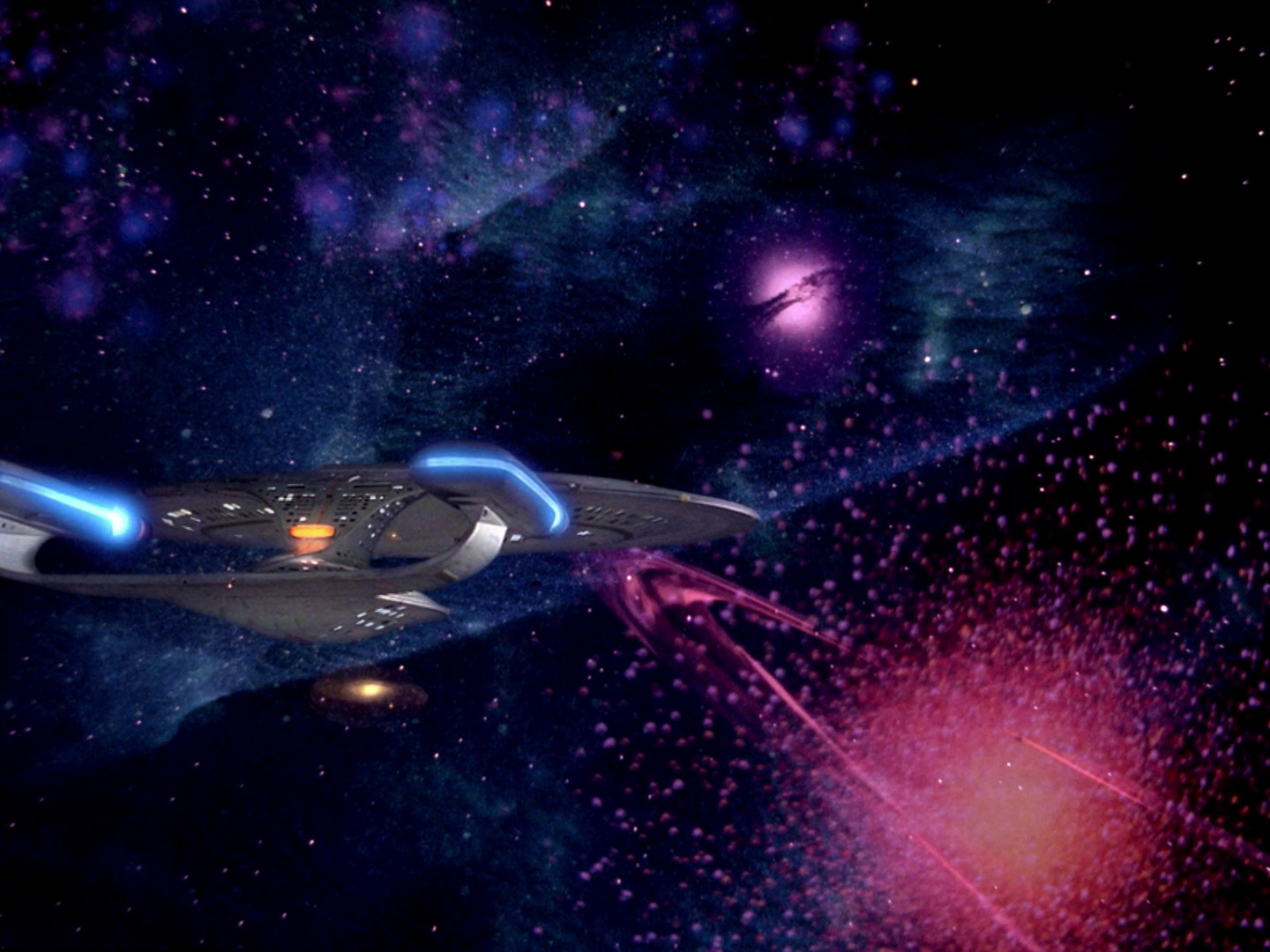Of course, the point is that, unlike the Sci-Fi franchise, the real velocity has never exceeded c, only the hyperbolic velocity and the proper velocity (note that in terms of SR, velocities approaching c imply very boosted frames, so despite we could travel to any point of the Universe in SR only approaching c very closely with respect to the traveler proper time-one human life-, but in terms of the “Earth” (or rest) reference frame millions of years would have passed away!).

When the coordinate-speeds approach c, the respective coordinate velocities deviate from this simple addition rule in that rapidities (hyperbolic velocity angle boosts) add instead of velocities, i.e.. Coordinate velocities add non-linearly. And it is a well-tested consequence of the Special Theory of relativity.  For highly relativistic objects (i.e. those with momentum per unit mass much larger than light speed) the result of the coordinate-velocity expression  familiar from most textbooks is rather uninteresting since the coordinate-velocities all peak out at c, i.e., as everybody knows, in special relativity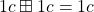, because applying the relativistic addition of velocities rule, we get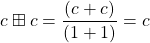And it is a fact from both theory and experiment! It will remain as long as SR remains a valid theory. SR holds yet with an astonishing degree of precision and accuracy. So, you can not deny every data and experiment that confirms SR. That is completely nonsense but there are some people and pseudo-scientists out there building their own theories AGAINST the achievements and explanations that SR provides to every experiment we have done until the current time. I am sorry for all of them. They are totally wrong. Science is not what they say it is. Any theory going beyond SR HAS to explain every experiment and data that SR does explain, and it is not easy to build such a theory or to say, e.g., why we have not observed (apparently) superluminal objects. I will discuss more superluminal in a forthcoming post/log entry, some posts after the special 50th post/log that is coming after this one! Stay tuned!

Coming back to our discussion…Why is all this stuff important? High Energy Physics is the natural domain of SR! And there, SR has not provided ANY wrong result till, in spite that some researches going beyond the Standard Model include modified dispersion relationships that reduce to SR in the low energy regime, we have not seen yet ANY deviation from SR until now.

For unidirectional motion, at low speeds the coordinate velocityof object 1 from the point of view of oncoming object 3 might be described as the sum of the velocity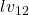of object 1 with respect to lab frame 2 plus the velocityof the lab frame 2 with respect to object 3, that is: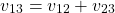Compare this expression to the previously obtained expression for rapidities! Rapidities always add, coordinate velocities add (linearly) only at low velocities. In conclusion, you must be careful by what you mean by velocity is a boosted system!

By the other hand, for relative proper-velocity, the result is:This expression shows how the momentum per unit mass as well as the map-distance traveled per unit traveler time of object 1, as seen in the frame of oncoming particle 3, goes as the sum of the coordinate-velocities times the product of the gamma (energy) factors. The proper velocity equation is especially important in high energy physics, because colliders enable one to explore proper-speed and energy ranges much higher than accessible with fixed-target collisions. For instance each of two electrons (traveling with frames 1 and 3) in a head-on collision traveling in the lab frame (2) at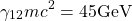or equivalenty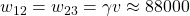lightseconds per traveler second  would see the other coming toward them at coordinate velocityandlightseconds per traveler second or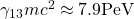. From the target’s view, that is an incredible increase in both energy and momentum per unit of mass.

Other magnitudes and their frame dependence in SR can be read from the following table: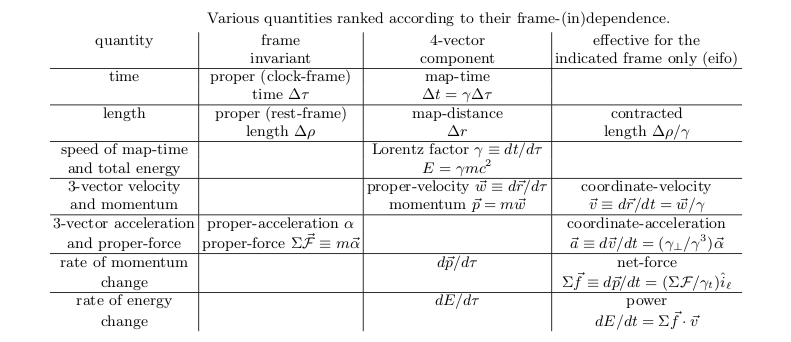CAUTION: These results don’t mean that the “real” energy is that. Energy is relative and it depends on the frame! The fact that in colliders, seen from the target reference frame, the energy can be greater than the center of mass energy is not an accident. It is a consequence of the formalism of special relativity. A similar observation can be done for velocities. Coordinate velocities, IN THE FRAMEWORK OF SPECIAL RELATIVITY, can never exceed the speed of light. As long as SR holds, there is no particle whose COORDINATE velocity can overcome the speed of light. However, we have seen that PROPER velocities are other monsters. They serve as a tool to handle rotations along the temporal axis, i.e., to handle boosts mixing space and time coordinates. Proper (or hyperbolic) velocities CAN be greater than speed of light. But, it does not contradict the special theory of relativity at all since hyperbolic velocities ARE NOT REAL since they are imaginary quantities and they are not physical. We can only measure momentum and real quantities!  Moreover, remember that, in fact, group or phase velocities we have found before can ALSO be greater than c. So, you must be careful by what do you mean by velocity in SR or in any theory. Furthermore, you must distinguish the notion of particle velocity with those of the relative velocity between two inertial frames, since the particle velocities ( coordinate or proper) always refer to some concrete frame! In summary, be aware of people saying that there are superluminal particles in our colliders or astrophysical processes. It is simply not true. Superluminal objects have observable consequences, and they have failed to be observed ( the last example was the superluminal neutrino affair by the OPERA collaboration, now in agreement with SR).

Remark (I): From the last table we observe that in SR, the rotation angle is imaginary. Therefore, we are forced to use this gadget of hyperbolic velocity in order to avoid “imaginary velocities”.

Remark (II): Hyperbolic velocities would become imaginary velocities if we used the imaginary formalism of SR, the infamous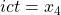.

Remark (III): Hyperbolic velocities are not coordinate velocities, so they are not physical at all. They are just a tool to provide the right answers in terms of rapidities, or the hyperbolic angle, whose units are imaginary radians! Hyperbolic velocities are measured in imaginary units of velocity!

Remark (IV): About the imaginary issues you can have now. The spacetime separation formulameans that the time t can often be treated mathematically as if it were an imaginary spatial dimension. That is, you can defineso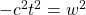, whereis the square root of  -1, andis a “fourth spatial coordinate”. Of course it is not at all. It is only a trick to treat the problem in a clever way.  By the other hand, a Lorentz boost by a velocity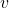can likewise be treated as a rotation by an imaginary angle. Consider a normal spatial rotation in which a primed frame is rotated in the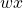-plane clockwise by an angle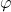about the origin, relative to the unprimed frame. The relation between the coordinates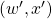and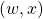of a point in the two frames is: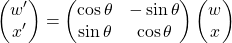Now setand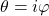, with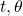both real. In other words, take the spatial coordinateto be imaginary, and the rotation anglelikewise to be imaginary. Then the rotation formula above becomes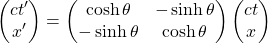This agrees with the usual Lorentz transformation formulae if the boost velocityand boost angleare related by the known formula. We realize that if we identify the imaginary angle with the rapidity, we are back to Special Relativity. Indeed, it is only the rotations involving the time axis which can cause confusion because they are so different from our everyday experience. That is, we experience rotations along some direction in our daily experience, so we are familiarized with rotations and their (real) rotation angles. However, rotations along a time axis mixing space and time is a weird creature. It uses imaginary numbers or, if we avoid them, we have to use hyperbolic (pseudo)-rotations.

SUMMARY OF MAIN IDEAS

A) Lorentz factorB) Proper-velocity or momentum per unit mass.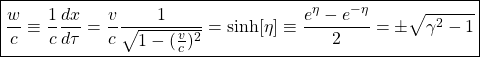C) Coordinate velocity.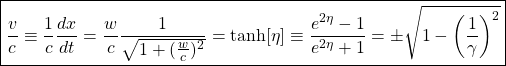D) Hyperbolic velocity angle or rapidity.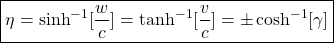or in terms of logarithms:E) Warp speed (just for fun):This site uses Akismet to reduce spam. Learn how your comment data is processed.